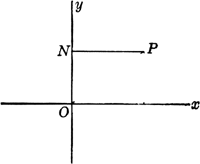# abscissa

(redirected from abscissae)
Also found in: Dictionary, Thesaurus, Medical.
Related to abscissae: ordinate

## abscissa:

see Cartesian coordinatesCartesian coordinates
[for René Descartes], system for representing the relative positions of points in a plane or in space. In a plane, the point P is specified by the pair of numbers (x,y
.

## abscissa

[ab′sis·ə]
(mathematics)
One of the coordinates of a two-dimensional coordinate system, usually the horizontal coordinate, denoted by x.

## abscissaabscissa: P, any point; NP, abscissa
In the plane Cartesian coordinate system, the horizontal coordinate of a point on a plane; the x-coordinate, obtained by measuring the distance from the point to the y-axis along a line parallel to the x-axis.

## abscissa

the horizontal or x-coordinate of a point in a two-dimensional system of Cartesian coordinates. It is the distance from the y-axis measured parallel to the x-axis

## abscissa

(mathematics)
The horizontal or x coordinate on an (x, y) graph; the input of a function against which the output is plotted.

The vertical or y coordinate is the "ordinate".

See Cartesian coordinates.
References in periodicals archive ?
Our approach can fail only if no set of m collocation abscissae can be found that results in a nonsingular matrix W.
[W.sub.1] = [1 0 0 0 0 0 0] [1 0 0 0 1 1 1] [1 1 1 1 0 0 0] [0 0 0 0 1 0 0] [0 0 0 0 1 2 3] [0 1 0 0 0 0 0] [0 1 2 3 0 0 0] Before discussing how to select the m collocation abscissae it is instructive to determine the maximum number of independent linear equations one can obtain using only the collocation constraints (9).
where [[Lambda].sub.max] is the desired new peak wavelength, while [Lambda][prime] and [[Lambda][prime].sub.max] are the corresponding abscissae of the template.
fx(z) [arrow left] MIR (fx(z), fxmin, fxmax) (4.1) fy(z) [arrow left] MIR (fy(z), fymin, fymax) (4.2) [n.sub.i,j + 1] [arrow left] MIR ([n.sub.i,j + 1,] Nmin, Nmax) (4.3) [Y.sub.i,j + 1] [arrow left] MIR ([Y.sub.i,j + 1,] [UPSILON]min, [UPSILON]max) (4.4) where fxmin, fxmax are the limits of the stochastic value that is added to the abscissae; fymin, fymax are the limits of the stochastic value that is added to the ordinates; Nmin, Nmax are the minimum and maximum numbers of samples per segment; and [UPSILON]min, [UPSILON]max are the minimum and maximum values of the ordinates.
Figures 5 and 6 refer to the first series of tests (cycle of equal mixing time) and they reproduce the temperature and power charts with the usual reference scales: on the abscissae (1 cm = 30 secs) and on the ordinates (1 cm = 25 [degrees] C and 200 kW).

Site: Follow: Share:
Open / Close